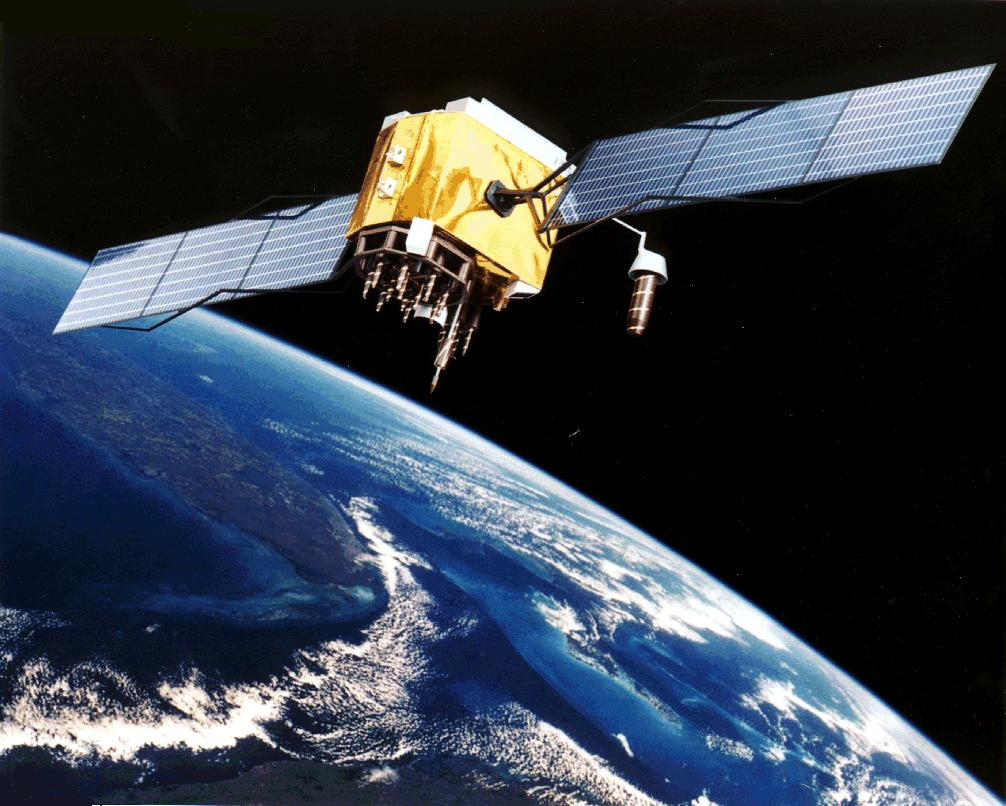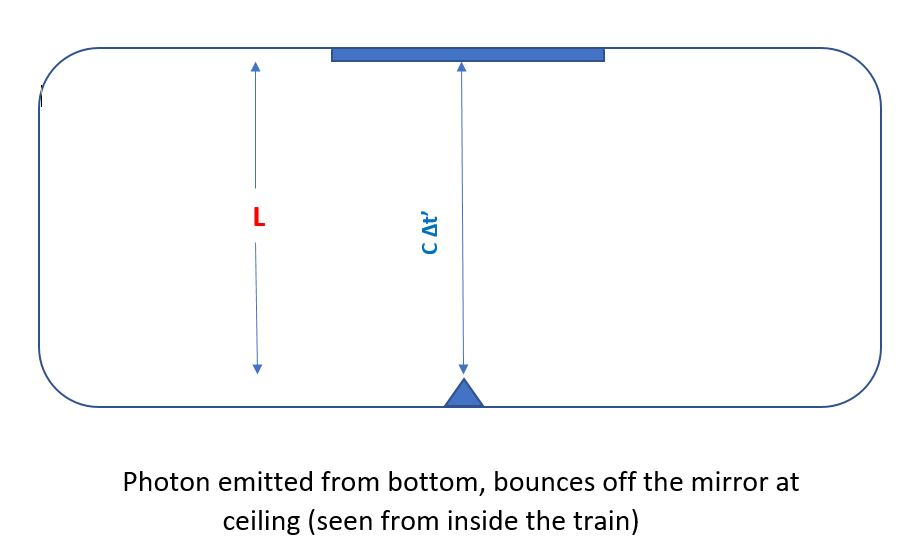# Mr. Einstein and my GPSI promised to continue one of my previous posts and explain how Einstein’s theories of 1905 and 1915 together affect our GPS systems. If we hadn’t discovered relativity (special and general) by now, we’d have certainly discovered it by the odd behaviour of our clocks on the surface of the earth and on an orbiting satellite.

The previous post ended by demonstrating that the time interval between successive ticks of a clock at the earth’s surface$\Delta t_R$ and a clock ticking infinitely far away from all masses$\Delta t_{\infty}$ are related by the formula$\Delta t_{R} = \Delta t_{\infty} (1 + \frac{ \Phi(R)}{c^2})$

The gravitational potential$\Phi(R)=-\frac{G M_E}{R}$ is a${\bf {negative}}$ number for all$R$. This means that the time intervals measured by the clock at the earth’s surface is${\bf {shorter}}$ than the time interval measured far away from the earth. If you saw the movie “Interstellar“, you will hopefully remember that a year passed on Miller’s planet (the one with the huge tidal waves) while 23 years passed on the Earth, since Miller’s planet was close to the giant Black Hole Gargantua. So time appears to slow down on the surface of the Earth compared to a clock placed far away.

Time for some computations. The mass of the earth  is$5.97 \times 10^{24} \: kg$, Earth’s radius is$R = 6370 \: km \: = 6.37\times 10^6 \: meters$ and the MKS units for$G = 6.67 \times 10^{-11} \: MKS \: units$. In addition, the speed of light$c = 3 \times 10^8 \frac {m}{s}$. If$\Delta t_{\infty} = 1 \: sec$, the clock on an orbiting satellite (assumed to be really far away from the earth) measures one second, the clock at the surface measures$\Delta t_R = (1 \: sec) \times (1 - \frac {(6.67 \times 10^{-11}) \: \times \: (5.97 \times 10^{-24} )}{(6.37 \times 10^6 )\: (3 \times 10^8 )^2})$

this can be simplified to$0.69 \: nanoseconds$ less than$1 \: sec$. In a day, which is$(24 \times 3600) \: secs$, this is$70 \times 10^-6 = 60 \: \mu \: seconds$ (microseconds are a millionth of a second).

In reality, as will be explained below, the GPS satellites are operating at roughly$22,000 \: km$ above the earth’s surface, so what’s relevant is the${\bf {difference}}$ in the gravitational potential at$28,370 \: km$ and$6,370 \: km$ from the earth’s center. That modifies the difference in clock rates to$53 \: nanoseconds$ per second, or$46 \: microseconds$ in a day.

How does GPS work? The US (other countries too – Russia, the EU, China, India) launched several satellites into a distant orbit$20,000 \: - 25,000 \: km$ above the earth’s surface. Most of the orbits are designed to allow different satellites to cover the earth’s surface at various points of time. A few of the systems (in particular, India’s) have satellites placed in a Geo-Stationary orbit, so they rotate around the earth with the earth – they are always above a certain point on the earth’s surface. The key is that they possess rather accurate and synchronized atomic clocks and send the time signals, along with the satellite position and ID to GPS receivers.

If you think about how to locate someone on the earth, if I told you I was 10 miles from the Empire State Building in Manhattan, you wouldn’t know where I was. Then, if I told you that I was 5 miles from the Chrysler building (also in Manhattan), you would be better off, but you still wouldn’t know how high I was. If I receive a third coordinate (distance from yet another landmark), I’d be set.  So we need distances from three well-known locations in order to locate ourselves on the Earth’s surface.

The GPS receiver on your dashboard receives signals from three GPS satellites. It knows how far they are, because it knows when the signals were emitted, as well as what the time at your location is.  Since these signals travel at the speed of light (and this is sometimes a problem if you have atmospheric interference), the receiver can compute how far away the satellites are. Since it has distances to three “landmarks”, it can be programmed to compute its own location.

Of course, if its clock was constantly running slower than the satellite clocks, it would constantly overestimate the distance to these satellites, for it would think the signals were$emitted \: earlier$ than they actually were. This would screw up the location calculation, to the distance travelled by light in$0.53 \: nanoseconds$, which is$0.16$ meters. Over a day, this would become$14$ kilometers. You could well be in a different city!

There’s another effect – that of time dilation. To explain this, there is no better than the below thought experiment, that I think I first heard of from George Gamow’s book. As with${\bf {ALL}}$ arguments in special and general relativity, the only things observers can agree on is the speed of light and (hence) the order of causally related events. That’s what we use in the below.

There’s an observer standing in a much–abused rail carriage. The rail carriage is travelling to the right, at a high speed$V$. The observer has a rather cool contraption / clock. It is made with a laser, that emits photons and a mirror, that reflects them. The laser emits photons from the bottom of the carriage towards the ceiling, where the mirror is mounted. The mirror reflects the photon back to the floor of the car, where it is received by a photo-detector (yet another thing that Einstein first explained!).The time taken for this up- and down- journey (the emitter and mirror are separated by a length$L$) is$\Delta t' = \frac{2 L}{c}$

That’s what the observer on the train measures the time interval to be. What does an observer on the track, outside the train, see?She sees the light traverse the path down in blue above. However, she also sees the light traveling at the same (numerical) speed, so she decides that the time between emission and reception of the photon is found using Pythagoras’ theorem$L^2 = (c \frac{\Delta t}{2})^2 - (V \frac {\Delta t}{2})^2$$\rightarrow \Delta t = \frac {2 L}{c} \frac{1}{\sqrt{1 - \frac{V^2}{c^2}}}$

So, the time interval between the same two events is computed to be larger on the stationary observer’s clock, than on the moving observer’s clock. The relationship is$\Delta t = \frac {\Delta t'}{ \sqrt{1 - \frac{V^2}{c^2}} }$

How about that old chestnut – well, isn’t the observer on the track moving relative to the observer on the train? How come you can’t reverse this argument?

The answer is – who’s going to have to turn the train around and sheepishly come back after this silly experiment runs its course? Well! The point is that one of these observers has to actively come back in order to compare clocks. Relativity just observes that you cannot make statements about${\bf {absolute}}$ motion. You certainly have to accept relative motion and in particular, how observers have to compare clocks at the same point in space.

From the above,$1$ second on the moving clock would correspond to$\frac {1}{ \sqrt{1 - \frac{V^2}{c^2}} }$ seconds on the clock by the tracks. A satellite at a distance$D$ from the center of the earth has an orbital speed of$\sqrt {\frac {G M_E}{D} }$, which for an orbit$22,000$ km above the earth’s surface, which is$28,370 \: km$ from the earth’s center, would be roughly$\sqrt { \frac {(6.67 \times 10^{-11} (5.97 \times 10^{-24})}{28370 \times 10^3} }\equiv 3700 \: \frac{meters}{sec}$

which means that$1$ second on the moving clock would correspond to$1 \: sec + 0.078 \: nanoseconds$ on the clock by the tracks. Over a day, this would correspond to a drift of$6 \: microseconds$, in the${\bf {opposite}}$ direction to the above calculation for gravitational slowing.

Net result – the satellite clocks run faster by$40$ microseconds in a day. They need to be continually adjusted to bring them in sync with earth-based clocks.

So, that’s three ways in which Mr. Einstein matters to you EVERY day!